Next: Newmann Problem in Spherical Up: Potential Theory Previous: Axisymmetric Charge Distributions

# Dirichlet Problem in Spherical Coordinates

We saw in Section 2.10 that the solution to the Dirichlet problem, in which the charge density is specified within some volume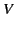, and the potential given on the bounding surface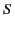, takes the form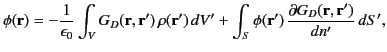(371)

where the Dirichlet Green's function is written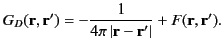(372)

Here,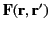is solution of Laplace's equation (i.e.,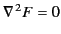) which is chosen so as to ensure that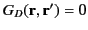when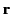(or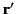) lies on. Thus, it follows from Sections 3.4 and 3.5 that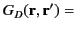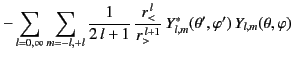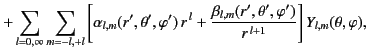(373)

where the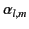and the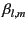are chosen in such a manner that the Green's function is zero whenlies on.

As a specific example, suppose that the volumelies between the two spherical surfaces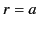and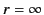. The constraint that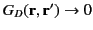as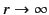implies that theare all zero. On the other hand, the constraintwhenyields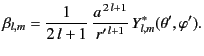(374)

Hence, the unique Green's function for the problem becomes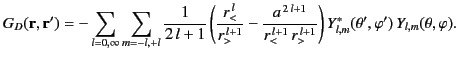(375)

Furthermore, it is readily demonstrated that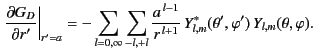(376)

It is convenient to write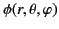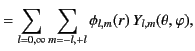(377)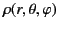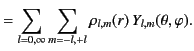(378)

It follows from Equation (311) that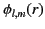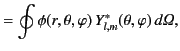(379)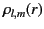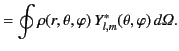(380)

Thus, Equations (372), (376) and (377) yield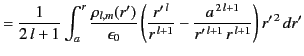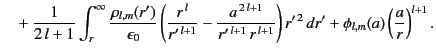(381)Next: Newmann Problem in Spherical Up: Potential Theory Previous: Axisymmetric Charge Distributions
Richard Fitzpatrick 2014-06-27